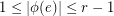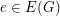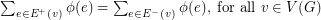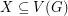Circular flow numbers of $r$-graphs

 Importance: Medium ✭✭
 Author(s): Steffen, Eckhard
 Subject: Graph Theory
 Keywords: flow conjectures nowhere-zero flows
 Posted by: Eckhard Steffen on: August 6th, 2015

A nowhere-zero-flowonis an orientationoftogether with a functionfrom the edge set ofinto the real numbers such that, for all, and.

A-regular graphis a-graph iffor everywithodd.

Conjecture   Letbe an integer. Ifis a-graph, then.

Since every-regular class 1 graph is a-graph, the truth of this conjecture would imply the truth of the conjecture on the circular flow number of regular class 1 graphs. If it is true for even, say, then Jaeger's modular orientation conjecture is true for-regular graphs and hence, by a result of Jaeger, it would imply the truth of Tutte's 5-flow conjecture. Forit is Tutte's 3-flow conjecture.

Bibliography

*[ES_2015]E. Steffen, Edge-colorings and circular flow numbers on regular graphs, J. Graph Theory 79, 1–7, 2015

* indicates original appearance(s) of problem.